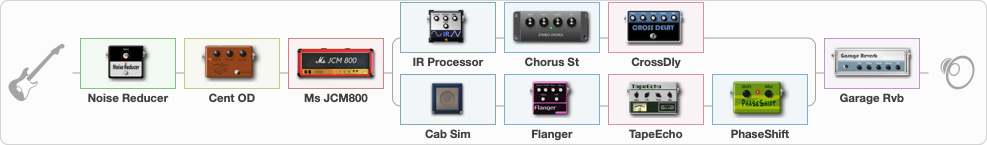# VH -- Little Dreamer -- Pananma

Discussion in 'ToneLib-GFX presets' started by davidtaylors, Apr 12, 2020.

1. ### davidtaylorsWell-Known Member

VH -- Little Dreamer -- Pananma

Preset name: VH Old School

Related video:

Effects chain:Effect: "Noise Reducer" (Dynamics / Filter), active - "yes"
{
"Sens" = 75
"Mode" = Soft
}

Effect: "Cent OD" (Overdrive / Distortion), active - "yes"
{
"Drive" = 91
"Tone" = 91
"Level" = 83
}

Effect: "Ms JCM800" (Amp simulators), active - "yes"
{
"Gain" = 46
"Bass" = 29
"Middle" = 73
"Treble" = 75
"Presence" = 65
"Master" = 29
"Level (dB)" = 2
}

Effect: "Splitter" (Dynamics / Filter), active - "yes"
{
"A-Bypass" = Off
"A-Pan" = -42
"A-Level" = 55
"B-Bypass" = Off
"B-Pan" = 50
"B-Level" = 55

'A' branch:
{

Effect: "IR Processor" (Cabinets), active - "yes"
{
"IR" = Cab IR Sample1
"Low Cut (Hz)" = 0
"Hi Cut (kHz)" = 20.0
"Mix" = 88
"Level (dB)" = 11
}

Effect: "Chorus St" (Modulation / Sfx), active - "yes"
{
"Speed" = 1.9
"Depth" = 31
"Center" = 4.0
"Mix" = 65
}

Effect: "CrossDly" (Delay), active - "yes"
{
"Time" = 756
"Feedback" = 27
"Tone" = 57
"Sens" = 29
"Mix" = 34
}
}
'B' branch:
{

Effect: "Cab Sim" (Cabinets), active - "yes"
{
"Model" = 4x12" Bluegrill
"Level (dB)" = 6
}

Effect: "Flanger" (Modulation / Sfx), active - "yes"
{
"Speed" = 0.8
"Depth" = 25
"Reso" = 61
"Center" = 8.1
"Offset" = 6.1
"Mix" = 15
"Reset" = On
}

Effect: "TapeEcho" (Delay), active - "yes"
{
"Time" = 257
"Feedback" = 73
"Tone" = 65
"LoDamp" = 75
"Mix" = 81
}

Effect: "PhaseShift" (Modulation / Sfx), active - "yes"
{
"Shift" = 74
"Mix" = 66
}
}
}

Effect: "Garage Rvb" (Reverb), active - "yes"
{
"Time" = 2.2
"PreDelay" = 56
"LoDamp" = 0
"HiDamp" = 30
"Mix" = 64
}

Note: You will need to download and install the ToneLib-GFX software to use the preset.

#### Attached Files:

• ###### VH_--_Little_Dreamer_--_Pananma.tlgfx
File size:
5.2 KB
Views:
3,847
Evgeniy ESP, Valera03, MPM and 8 others like this.